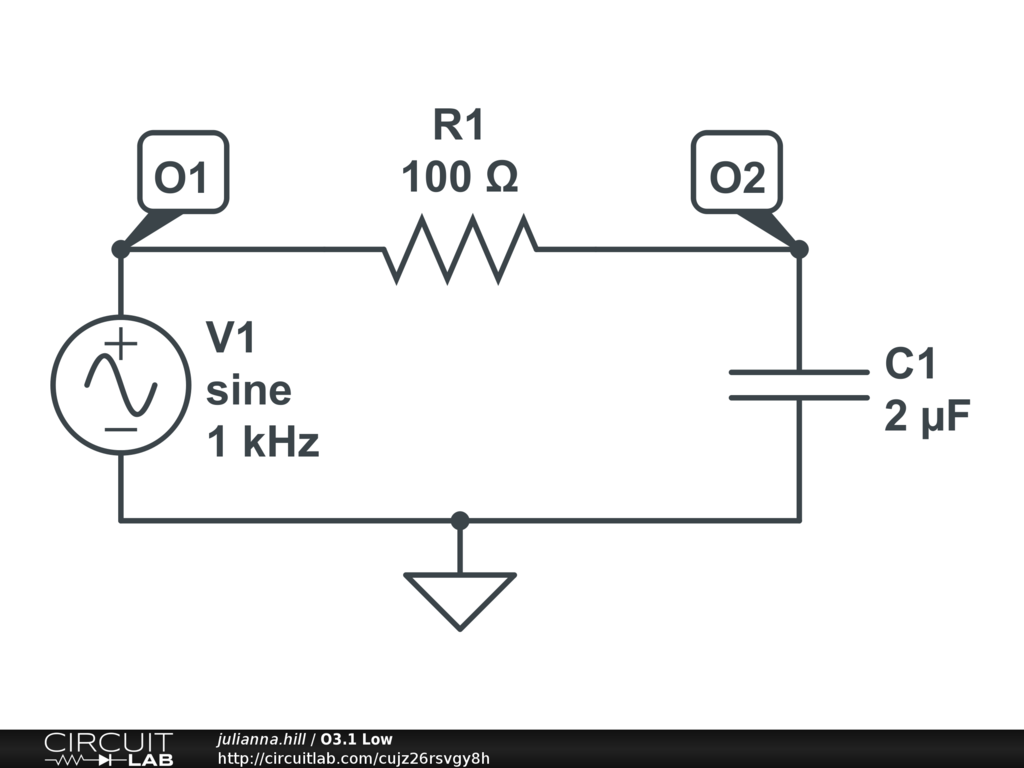## dB Log Scale BugSOLVED

 I am trying to plot the gain of a low-pass filter on a log scale, but the graph simply doesn't appear when I put it in log scale, but it works with linear.Here I have tried to graph one function on a linear scale successfully, and another on a third plot with log scale, which simply does not appear. by julianna.hill May 13, 2020 Hi @julianna.hill, on your third graph, you are plotting DB(MAG(V(O1))) in a frequency-domain simulation, and you have it configured to use a logarithmic scale on the y-axis. The issue is that you're basically trying to plot a logarithm of a logarithm, and the inner log is 0. Since log(0) = -infinity, it's unable to plot. The first log is happening within your expression itself: DB(1) = 0 . So, perhaps counterintuitively, you should actually be using a Linear y-axis scale when plotting values in decibels. That's because you want equal spacing between -60,-40,-20,0,+20 dB etc -- that's just a linear scale. Does that help? by mrobbins May 13, 2020 I see, I didn't realize it was for the y-axis! My professor has asked us to plot dB as a function of log(f) (i.e. with the x-axis on log scale). Is this possible in circuitlab? by julianna.hill May 13, 2020 Yes, a logarithmic x-axis scale is actually the default and only option when doing frequency-domain simulation! If you open your existing circuit and run the simulation, you'll see that 10Hz, 100Hz, 1kHz, 10kHz are equally spaced on the x-axis of all of your plots. This is already a logarithmic scale. If you need to explicitly show the base-10 logarithm on the x-axis labels, my recommendation would be to click "Export Plot CSV" and then take your raw simulation data into a different program to create whatever plot you'd like. by mrobbins May 13, 2020 Ahaha my mistake, thank you very much! by julianna.hill May 13, 2020